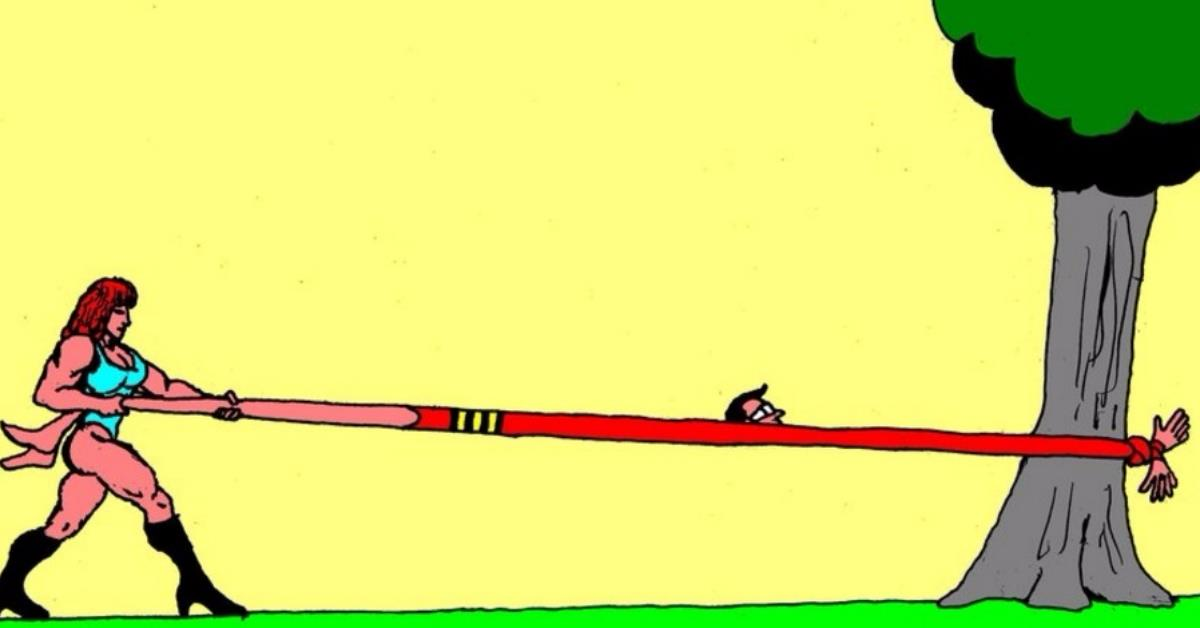# Price Elasticity of DemandUpdated on Wednesday, May 03, 2017

,tags  #  # #

The price elasticity of demand, Ed is defined as:

Ed= (proportionate change in quantity demanded )/(proportionate change in price)

Since the quantity demanded decreases when the price increases, this ratio is negative; however, the absolute value usually is taken and Ed is reported as a positive number.
Because the calculation uses proportionate changes, the result is a unitless number and does not depend on the units in which the price and quantity are expressed.
As an example calculation, take the case in which a product's Ed is reported to be 0.5. Then, if the price were to increase by 10%, one would observe a decrease of approximately 5% in quantity demanded.
In the above example, we used the word "approximately" because the exact result depends on whether the initial point or the final point is used in the calculation. This matters because for a linear demand curve the price elasticity varies as one moves along the curve. For small changes in price and quantity the difference between the two results often is negligible, but for large changes the difference may be more significant. To deal with this issue, one can define the arc price elasticity of demand. The arc elasticity uses the average of the initial and final quantities and the average of the initial and final prices when calculating the proportionate change in each. Mathematically, the arc price elasticity of demand is defined as:Q2 - Q1
-----------------------
( Q1 + Q2 ) / 2
-------------------------------
P2 - P1
-----------------------
( P1 + P2 ) / 2
where
Q1=Initial quantity
Q2=Final quantity
P1=Initial price
P2=Final price

## Elastic versus Inelastic

E  > 1
In this case, the quantity demanded is relatively elastic, meaning that a price change will cause an even larger change in quantity demanded. The case of Ed = infinity is referred to as perfectly elastic. In this theoretical case, the demand curve would be horizontal. For products having a high price elasticity of demand, a price increase will result in a revenue decrease since the revenue lost from the resulting decrease in quantity sold is more than the revenue gained from the price increase.
E  < 1
In this case, the quantity demanded is relatively inelastic, meaning that a price change will cause less of a change in quantity demanded. The case of Ed = 0 is referred to as perfectly inelastic. In this theoretical case, the demand curve would be vertical. For products whose quantity demanded is inelastic, a price increase will result in a revenue increase since the revenue lost by the relatively small decrease in quantity is less than the revenue gained from the higher price.
E  = 1
In this case, the product is said to have unitary elasticity; small changes in price do not affect the total revenue.

## Factors Affecting the Price Elasticity of Demand

• Availability of substitutes: the more possible substitutes, the greater the elasticity. Note that the number of substitutes depends on how broadly one defines the product.
• Degree of necessity or luxury: luxury products tend to have greater elasticity. Some products that initially have a low degree of necessity are habit forming and can become "necessities" to some consumers.
• Proportion of the purchaser's budget consumed by the item: products that consume a large portion of the purchaser's budget tend to have greater elasticity.
• Time period considered: elasticity tends to be greater over the long run because consumers have more time to adjust their behavoir.
• Permanent or temporary price change: a one-day sale will elicit a different response than a permanent price decrease.
• Price points: decreasing the price from \$2.00 to \$1.99 may elicit a greater response than decreasing it from \$1.99 to \$1.98.